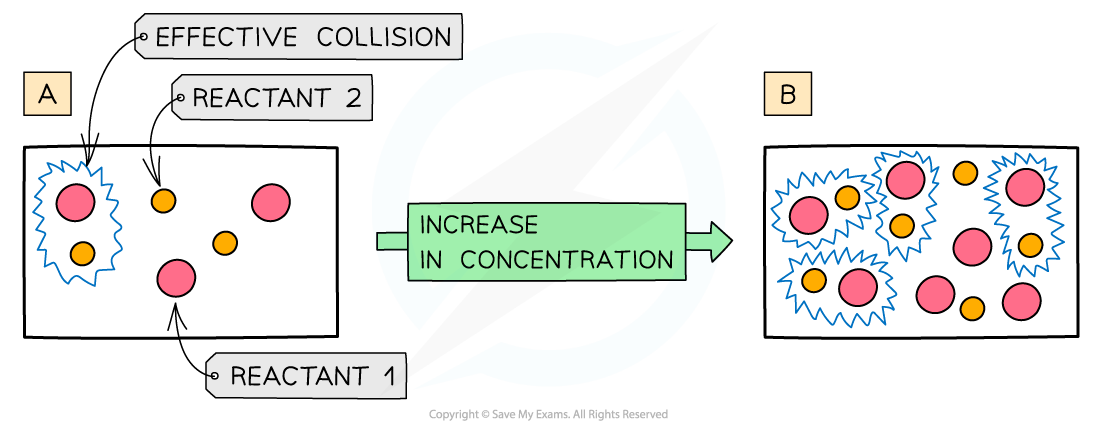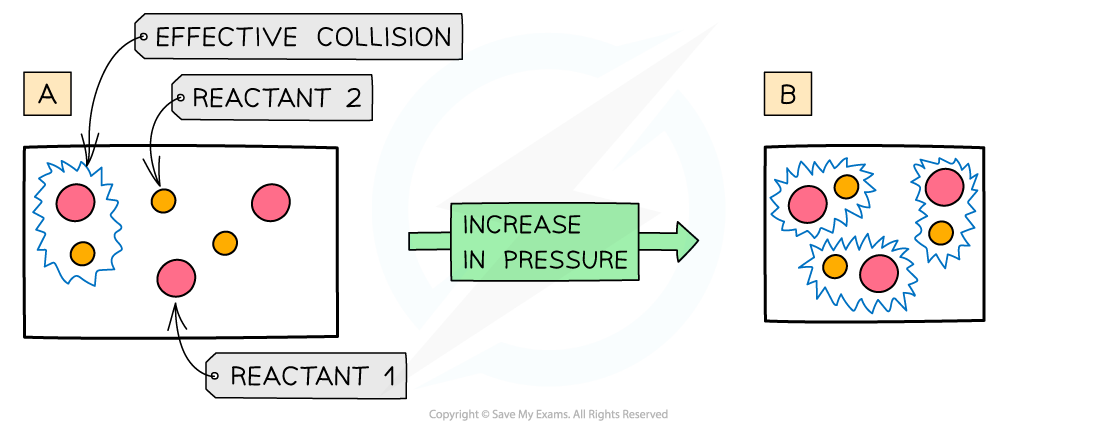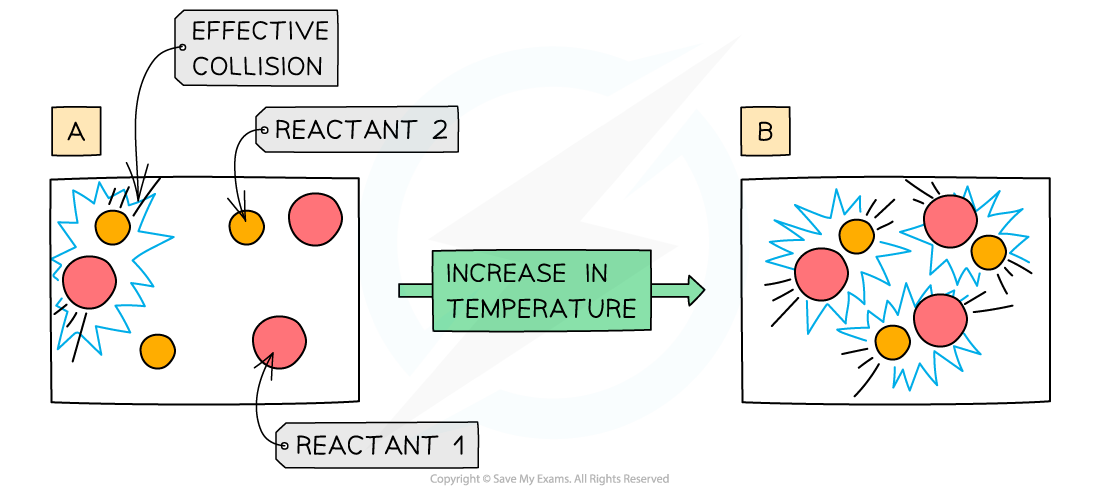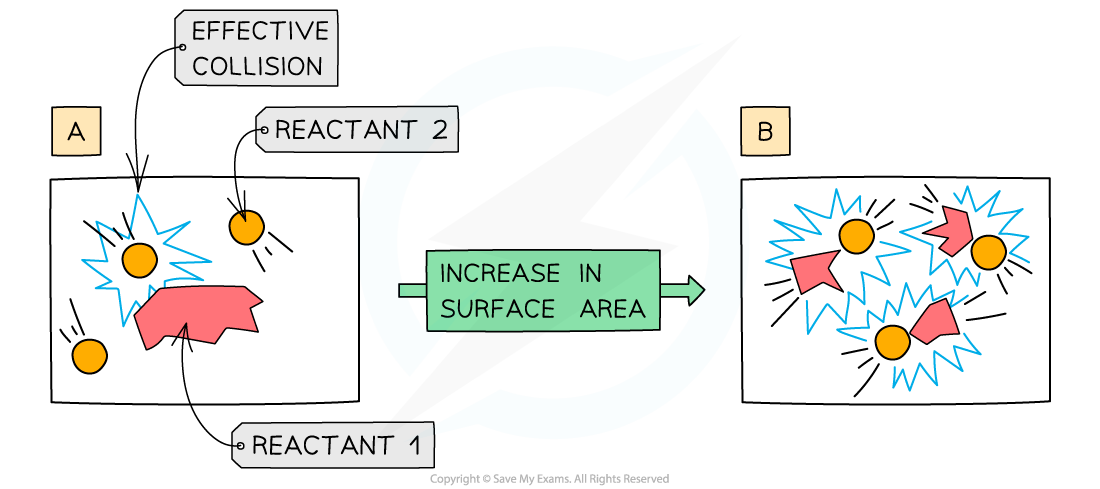# IB DP Chemistry: SL复习笔记6.1.6 Explaining Rates

### Explaining Rates

#### Concentration

• The more concentrated a solution is, the greater the number of particles in a given volume of solvent
• An increase in concentration causes in an increased collision frequency and therefore an increased rate of reactionThe diagram shows a higher concentration of particles in (b) which means that there are more particles present in the same volume than (a) so the chances and frequency of collisions between reacting particles is increased causing an increased rate of reaction

#### Pressure

• An increase in pressure in reactions that involve gases has the same effect as an increased concentration of solutions
• When the pressure is increased, the molecules have less space in which they can move
• This means that the number of effective collisions increases due to an increased collision frequency
• An increase in pressure therefore increases the rate of reactionThe diagram shows a higher pressure in (b) which means that the same number of particles occupy a smaller volume, resulting in an increased collision frequency and therefore increased rate of reaction

#### Temperature

• Increasing the temperature of the reaction mixture increases the rate of reaction in the following two ways:
• At higher temperatures, the particles are moving faster, so collide more frequently. A higher number of collisions in total mean a higher number of successful collisions
• At higher temperatures, a higher proportion of the molecules have the activation energy or more. This means that a higher proportion of collisions are successfulAn increase in temperature causes an increase in the kinetic energy of the particles. The number of collisions increases and the proportion of successful collisions increases

#### Particle Size

• Only the particles on the surface of a solid will collide with particles of the other reactant
• If the surface area is increased, more particles will be on the surface and able to collide with particles of the other reactant
• This means that there will be more collisions in total and therefore more successful collisions.
• Surface area can be increased by decreasing the size of the reactant particles
• For example, powders have a very large surface areaAn increase in surface area of a solid reactant allows more particles to come into contact with each other

#### Worked Example

Which of the following factors can result in the rate of a chemical reaction increasing?

I.  Increasing the total pressure in reactions involving gases

II.  Raising the temperature in reactions involving gases

III.  Increasing the particle size of a solid in a reaction

A.   I and II only

B.   I and III only

C.   II and III only

D.   I, II and III

The correct option is A.

• Increasing pressure and raising temperature increases the number of successful collisions
• Increasing the particle size decreases the surface area and reduces the number of collisions